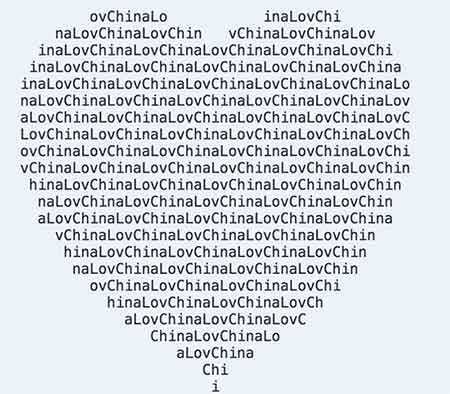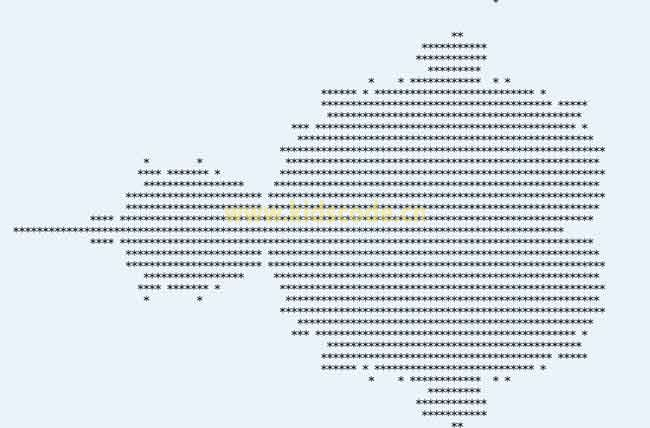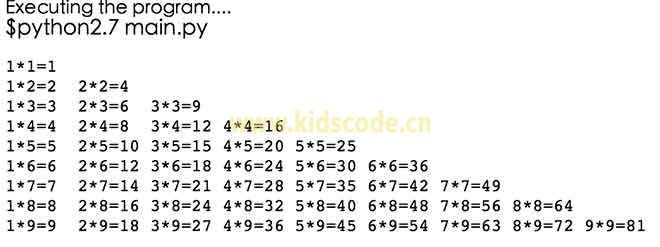經常會有小朋友問我，“我想做個黑客，我該學什么編程語言？”，或者有的小朋友會說：“我要學c，我要做病毒”。其實對于這些小朋友而言他們基本都沒有接觸過編程語言，只是通過影視或者其他地方看到的一些東西認為黑客很酷，超級厲害，因此也萌生了這樣的想法。我也認為黑客很厲害，他們不只是會一門編程語言那么簡單，要想成為他們那樣厲害的人對小朋友來說還有很長的路要走。

而很多小朋友真正想做的就是能通過簡單的代碼做出很酷炫的東西，能在同學間炫耀一把，那就很滿足了。如果你真的想學編程，那么我還是建議python，看到下面這些神奇的代碼，也許你會改變自己初的想法。不是我們覺得C不好（很多人是從c入門而從此心生畏懼，受挫而放棄的），而是python更適合入門，當你通過一段時間的學習，你真的愛上了編程，你可以再去學習c，這樣你之前所積累的知識會讓你對它有更深刻的理解與認知，畢竟興趣真的很重要。

如果你能在同學朋友面前秀一下這行代碼，我估計他們會用特別崇拜的眼神看你的。

print'\n'.join([''.join([('ChinaLove'[(x-y)%8]if((x*0.05)**2+(y*0.1)**2-1)**3-(x*0.05)**2*(y*0.1)**3<=0 else' ')for x in range(-30,30)])for y in range(15,-15,-1)])

在python 里執行它，會輸出一個字符拼出的心形，是不是很酷啊。再來一個，雖然你可能不知道這個東西，不過看看結果也是讓你很激動的。

有一個的圖像叫mandelbrot。Mandelbrot圖像中的每個位置都對應于公式N=x+y＊i中的一個復數，高中學過復數的都還應該有印象。每個位置用參數N來表示，它是x＊x+y＊y的平方根。如果這個值大于或等于2，則這個數字對應的位置值是 0。如果參數N的值小于2，就把N的值改為N*N-N（N=（x*x-y*y-x）+（2*x*y-y）*i）），并再次測試這個新N值。wiki百科給出的圖像是這樣的：讓我們用一行代碼畫一個Mandelbrot：

print'\n'.join([''.join(['*'if abs((lambda a:lambda z,c,n:a(a,z,c,n))(lambda s,z,c,n:z if n==0else s(s,z*z+c,c,n-1))(0,0.02*x+0.05j*y,40))<2 else' 'for x in range(-80,20)])for y in range(-20,20)])

``

對于隨手小工具而言，更是Python的拿手好戲。

一行代碼打印九九乘法表:

print '\n'.join([' '.join(['%s*%s=%-2s' % (y,x,x*y) for y in range(1,x+1)]) for x in range(1,10)])如何？如果你想學編程，我們首先推薦小朋友從scratch開始，生產型語言進階學習python是個不錯的選擇。